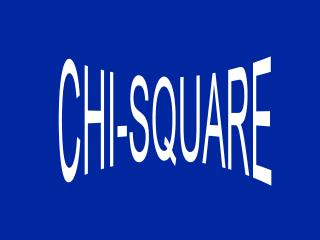DownloadDownload PresentationChi Square

# Chi Square

Download Presentation## Chi Square

- - - - - - - - - - - - - - - - - - - - - - - - - - - E N D - - - - - - - - - - - - - - - - - - - - - - - - - - -
##### Presentation Transcript

1. Chi Square • Chi square is employed to test the difference between an actual sample and another hypothetical or previously established distribution such as that which may be expected due to chance or probability. Chi square may also be used to test the differences between two or more actual samples.

2. Basic Computation Equation

3. Basic Computation Equation • With Yates Correction: Used for Problems With 1 Degree of Freedom

4. One Way Classification • This test is used to when a researcher is interested in the number of responses, objects, or people that fall into two or more categories. This procedure is sometimes called the “goodness-of-fit” statistic.

5. One Way Classification • Suppose a coin toss experiment involving twenty trials yielded 12 heads and 8 tails. Is this result significantly different from the expected frequency of 10 heads and 10 tails? • Observed Expected • Heads 12 10 • Tails 08 10

6. One Way Classification .225 .225 .450

7. Two Way Classification • The two-way chi square is a convenient technique for determining the significance of the difference between the frequencies of occurrence between two or more categories with two or more groups.

8. Calculating Degrees of Freedom • One independent variable - df = (r-1), where r equals the number of levels of the independent variable • Two independent variables - df = (r-1) (s-1), where r and s are the number of levels of the first and second independent variables, respectively • Three independent variables - df = (r-1) (s-1) (t-1), where r, s and t are the number of levels of the first, second and third independent variables, respectively.

9. Assumptions • The data is in frequency form. • The observations are independent of each other. • Distribution basis is decided before data collection. • The sum of observed and expected frequencies are equal. • Sample size is adequate. In a 2x2 table, chi square should not be used when n is less than 20. In a larger table, no cell should have an expected value of less than 1 and not more than 20% of the cells can have expected values of less than 5.